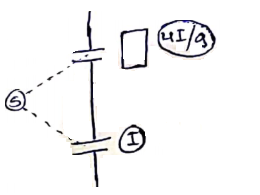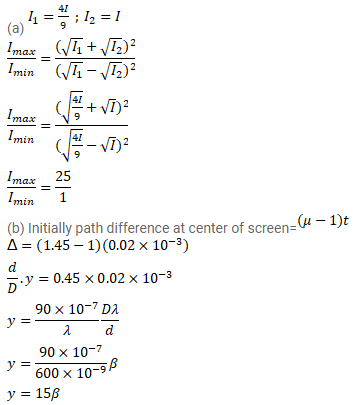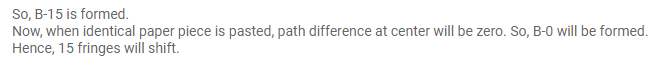# A thin paper of thickness 0.02 mm having a refractive index 1.45Question:

A thin paper of thickness $0.02 \mathrm{~mm}$ having a refractive index $1.45$ is pasted across one of the slits in a Young's double slit experiment. The paper transmits $4 / 9$ of the light energy falling on it. (a) Find the ratio of the maximum intensity to the minimum intensity in the fringe pattern. (b) How many fringes will cross through the centre if an identical paper piece is pasted on the other slit also? The wavelength of the light used is $600 \mathrm{~nm}$.

Solution: